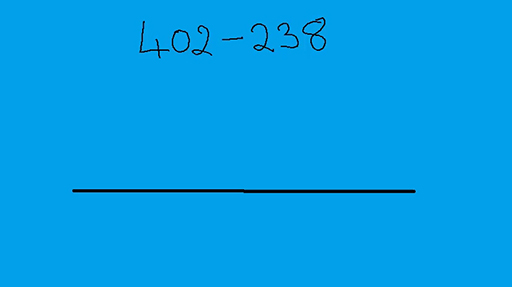### Become an OU studentTeaching mathematics

Start this free course now. Just create an account and sign in. Enrol and complete the course for a free statement of participation or digital badge if available.

# 2.2 Mental addition and subtraction strategies

Using number bonds can help with mental addition calculations because numbers can be split up to make the tens or hundreds number and then the extra can be added on.

## Activity 3 Adding large numbers mentally

Timing: Allow 5 minutes

Try these questions and reflect on your methods.

• a.

• b.

• c.

### Discussion

Did you partition the numbers into smaller numbers? For example, for question (a) did you work out 40 + 30 and then work out 3 + 2?

Did you bridge across a multiple of ten? For example, in question (b) did you mentally change 47 + 38 into 47 + 3 (to make 50) and then add 35?

Once a learner can calculate what to add to 38 to make 100 they can answer the question 100 – 38 and move on to calculations such as 402 – 238 by bridging through 300.

This mental arithmetic can be illustrated using an empty number line. The actions on the number line can act as a way to record the stages in a mental calculation. This is shown in the following video.

Download this video clip.Video player: Video 1 Using the empty number line to subtract 238 from 402Video 1 Using the empty number line to subtract 238 from 402
Interactive feature not available in single page view (see it in standard view).

We can use this method to answer questions such as £4.02 – £2.38 or 4.03 m – 2.38 m, taking us into the realm of using measures. This method also helps with time calculations where learners need to remember that time is not a decimal system. For example, a train journey which starts at 2.38 pm and goes on until 4.02 pm takes 22 minutes + 1 hour + 2 minutes which comes to 1 hour 24 minutes (bridging through 3 pm and 4 pm).

## Activity 4 Subtraction problems for you to solve

Timing: Allow 5 minutes